# Black boots

This item is of boots class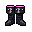You stub your toe on this pair of black boots. Perhaps they bring you bad luck?
Attributes
DEF
15
MIN_LEVEL
100

My level ...
Resistances
LIGHT
83%
DARK
111%
Dropped by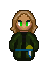assassin
Drops exactly 1.
Probability: 0.005%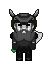black duergar
Drops exactly 1.
Probability: 0.001%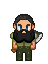mountain hero dwarf
Drops exactly 1.
Probability: 0.005%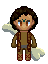barbarian king
Drops exactly 1.
Probability: 0.025%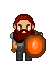mountain leader dwarf
Drops exactly 1.
Probability: 0.035%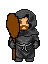emperor dalmung
Drops exactly 1.
Probability: 0.1%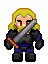blordrough corporal
Drops exactly 1.
Probability: 0.25%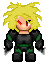blordrough storm trooper
Drops exactly 1.
Probability: 0.5%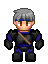blordrough elite
Drops exactly 1.
Probability: 0.5%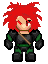blordrough infantry
Drops exactly 1.
Probability: 0.5%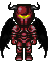chaos overlord
Drops exactly 1.
Probability: 0.1%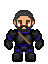blordrough captain
Drops exactly 1.
Probability: 0.5%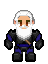blordrough general
Drops exactly 1.
Probability: 0.5%